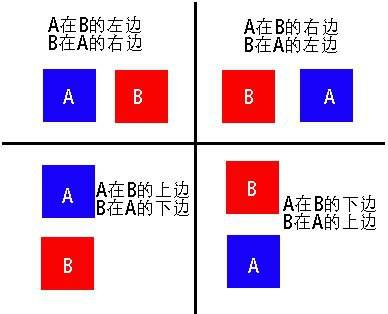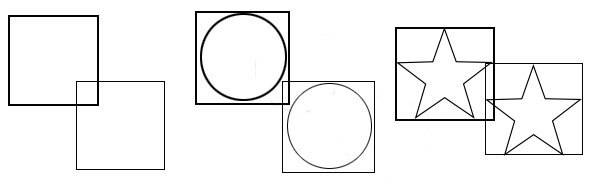# canvas动画包教不包会：碰撞检测

• 从几何图形的角度来检测，就是判断一个物体是否与另一个有重叠，我们可以用物体的矩形边界来判断。
• 检测距离，就是判断两个物体是否足够近到发生碰撞，需要计算距离和判断两个物体是否足够近。

1、基于几何图形的碰撞检测

1.1 两个物体间的碰撞检测（矩形边界检测法）

``function getBound(body){     return  {       x: (body.x - body.radius),       y: (body.y - body.radius),       width: body.radius * 2,       height: body.radius * 2     };   }``

``tool.intersects = function(bodyA,bodyB){  return !(bodyA.x + bodyA.width < bodyB.x ||          bodyB.x + bodyB.width < bodyA.x ||          bodyA.y + bodyA.height < bodyB.y ||          bodyB.y + bodyB.height < bodyA.y);};````if(tool.intersects(objectA,objectB)){  console.log('撞上了');}````if(activeRect !== rect && tool.intersects(activeRect, rect)) {     activeRect.y = rect.y - activeRect.height;     activeRect = createRect();   };``

1.2 物体与点的碰撞检测

``tool.containsPoint = function(body, x, y){     return !(x < body.x || x > (body.x + body.width)            || y < body.y || y > (body.y + body.height));  };``

``if(tool.containsPoint(body,50,50)){  console.log('在矩形内');}``

tool.intesects()和tool.containsPoint()方法都会遇到精确问题，对矩形最精确，越不规则，精确率就越小。大多数情况下，都会采取这两种方法。当然，如果你要对不规则图形采取更精确的方法，那你就要写更多的代码去执行精确的检测了。

2、基于距离的碰撞检测

2.1 基于距离的简单碰撞检测

``dx = ballB.x - ballA.x;dy = ballB.y - ballA.y;dist = Math.sqrt(dx * dx + dy * dy);if(dist < ballA.radius + ballB.radius){  console.log('碰撞了');}``

``var dx = ballB.x - ballA.x;   var dy = ballB.y - ballA.y;   var dist = Math.sqrt(dx * dx + dy * dy);   if(ball != ballB && dist < ballA.radius + ballB.radius){     ctx.strokeStyle = 'red';     var txt = '你压着我了';     var tx = ballA.x - ctx.measureText(txt).width / 2;     ctx.font = '30px Arial'     ctx.strokeText(txt,tx,ballA.y);   };``

2.2 弹性碰撞

3、多物体的碰撞检测策略

``for(var i = 0; i < objects.length; i++){  var objectA = objects[i];  for(var j = 0; j < objects.length; j++){    var objectB = objects[j];    if(tool.intersects(objectA,objectB){}  }};``

（1）多余的自身碰撞检测

``if(i != j && tool.intersects(objectA,objectB){}``

（2）重复碰撞检测

``for(var i = 0; i < objects.length; i++){  var objectA = objects[i];  for(var j = i + 1; j < objects.length; j++){    var objectB = objects[j];    if(tool.intersects(objectA,objectB){}  }};``

``function checkCollision(ballA, ballB) {     var dx = ballA.x - ballB.x;     var dy = ballA.y - ballB.y;     var dist = Math.sqrt(dx * dx + dy * dy);     var min_dist = ballB.radius + ballA.radius;     if(dist < min_dist) {       var angle = Math.atan2(dy, dx);       var tx = ballB.x + Math.cos(angle) * min_dist;       var ty = ballB.y + Math.sin(angle) * min_dist;       var ax = (tx - ballA.x) * spring * 0.5;       var ay = (ty - ballA.y) * spring * 0.5;       ballA.vx += ax;       ballA.vy += ay;       ballB.vx += (-ax);       ballB.vy += (-ay);     };   };``

（1）矩形边界碰撞检测

``tool.intersects = function(bodyA,bodyB){  return !(bodyA.x + bodyA.width < bodyB.x ||          bodyB.x + bodyB.width < bodyA.x ||          bodyA.y + bodyA.height < bodyB.y ||          bodyB.y + bodyB.height < bodyA.y);};``

（2）基于距离的碰撞检测

``dx = objectB.x - objectA.x;dy = objectB.y - objectA.y;dist = Math.sqrt(dx * dx + dy * dy);if(dist < objectA.radius + objectB.radius){}``

（3）多物体碰撞检测

``for(var i = 0; i < objects.length; i++){  var objectA = objects[i];  for(var j = i + 1; j < objects.length; j++){    var objectB = objects[j];    if(tool.intersects(objectA,objectB){}  }};``

App下载